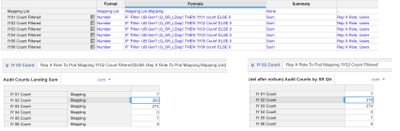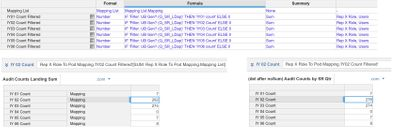# SUM results not matching up to summaryI'm having an issue with SUM giving me unexpected results.

When I'm working with hierarchy lists and just need a top level sum rather than subtotals at all levels of the hierarchy, sometimes I'll use a 1-item list, add a line item set to this list item, and SUM over it to get the top level sum. It's a little convoluted but saves space and calculations vs. turning on summary over all the intermediate hierarchy levels.

However, I just tried this and got a different number from the line item summary. While the line item summary gives me 276 at the top level (which is correct,) using the SUM function over my helper list item gives me 282.

I can't figure out why this is happening. Is there something I'm missing/forgetting about using SUM in this way?Tagged:

•Can you share some screenshots demonstrating this?

•The top module's dimension is "Rep X Role"

The lower left module is dimensionalized by Users + "Mapping List" and uses the SUM function, while lower right module is dimensionalized by "Users" and is pulling in the top level from the line item summary.

•Ok, it looks like you are doing it correctly and the numbers are correct except for IY02, so it must be somewhere in the data.  Could you be summing data (bottom left box) where it is not valid?

Not that you asked this, but since you did share the blueprint (yea, I know, I asked 🙂 ), you may want to consider using a line item subset so you are not repeating the line item formula so many times and so it can potentially scale if you ever create more IY filters.

Rob

•@rob_marshall what do you mean about data not being valid? Since I'm using the same line item in both cases, I assumed that any data issues that affected one way would affect the other, as long as the logic is correct.

Thanks for the tip about LIS - this is a "quick and dirty" proof of concept rework of an existing build. We will definitely clean it up once we are confident it's working correctly.

•I am thinking, and again without seeing the data, I am wondering if you are summing data for IY02 that you shouldn't which could be throwing off the count.  Since the top module has Users (the mapping line item), I am wondering if it is mapping a user to a value that shouldn't be there.  It would way easier to find if the number was not in the hundreds, but I would look at the bottom left module and make sure all 284 are "valid".  That is where I would start.

Rob